JMSLTM Numerical Library 7.2.0
com.imsl.math

Class Physical

• All Implemented Interfaces:
Serializable, Cloneable

public class Physical
extends Number
implements Serializable, Cloneable
Return the value of various mathematical and physical constants. The case of the String specifying the name of the physical constant does not matter. The names 'PI', 'Pi', 'pI' and 'pi' are equivalent. The units of the physical constants are in SI units, (meter-kilogram-second). The names allowed are as follows:

 Name Description Value Reference AMU Atomic mass unit 1.6605402E-27 kg  ATM Standard atm pressure 1.01325E+5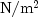E AU Astronomical unit 1.496E+11 m [ ] Avogadro Avogadro's number 6.0221367E+23 1/mole  Boltzman Boltzman's constant 1.380658E-23 J/K  C Speed of light 2.997924580E+8 m/s E Catalan Catalan's constant 0.915965... E E Base of natural logs 2.718... E ElectronCharge Electron change 1.60217733E-19 C  ElectronMass Electron mass 9.1093897E-31 kg  ElectronVolt Electron volt 1.60217733E-19 J  Euler Euler's constant gamma 0.577... E Faraday Faraday constant 9.6485309E+4 C/mole  FineStructure Fine structure 7.29735308E-3  Gamma Euler's constant 0.577... E Gas Gas constant 8.314510 J/mole/K  Gravity Gravitational constant 6.67259E-11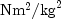Hbar Planck constant / 2 pi 1.05457266E-34 J*s  PerfectGasVolume Std vol ideal gas 2.241383E-2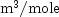[*] Pi Pi 3.141... E Planck Planck's constant h 6.6260755E-34 J*s  ProtonMass Proton mass 1.6726231E-27 kg  Rydberg Rydberg's constant 1.0973731534E+7 /m  SpeedLight Speed of light 2.997924580E+8 m/s E StandardGravity Standard g 9.80665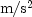E StandardPressure Standard atm pressure 1.01325E+5E StefanBoltzmann Stefan-Boltzman 5.67051E-8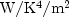WaterTriple Triple point of water 2.7316E+2 K E

The reference for constants are indicated by the code in the [] comment above.

  Cohen and Taylor (1986)  Liepman (1964)  Precomputed mathematical constants

The constants marked with an E before the [] are exact (to machine precision).

1. Units strings have the form U1*U2*...*Um/V1/.../Vn, where Ui and Vi are the names of basic units or are the names of basic units raised to a power. Examples are, 'METER*KILOGRAM/SECOND', 'M*KG/S', 'METER', or '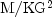'. These strings are case insensitive.
2. The basic unit names allowed are as follows.

Units of time
day, hour = hr, min = minute, s = sec = second, year

Units of frequency
Hertz = Hz

Units of mass
AMU, g = gram, lb = pound, ounce = oz, slug

Units of distance
Angstrom, AU, ft = feet = foot, in = inch, m = meter = metre, micron, mile, mill, parsec, yard

Units of area
acre

Units of volume
l = liter = litre

Units of force
dyne, N = Newton, poundal

Units of energy
BTU(thermochemical), Erg, J = Joule

Units of work
W = watt

Units of pressure
ATM = atomosphere, bar, Pascal

Units of temperature
degC = Celsius, degF = Fahrenheit, degK = Kelvin

Units of viscosity
poise, stoke

Units of charge
Abcoulomb, C = Coulomb, statcoulomb

Units of current
A = ampere, abampere, statampere

Units of voltage
Abvolt, V = volt

Units of magnetic induction
T = Tesla, Wb = Weber

Other units
1, farad, mole, Gauss, Henry, Maxwell, Ohm

The following metric prefixes may be used with the above units. Note that the one or two letter prefixes may only be used with one letter unit abbreviations.

A = atto = 1.E-18
F = femto = 1.E-15
P = pico = 1.E-12
N = nano = 1.E-9
U = micro = 1.E-6
M = milli = 1.E-3
C = centi = 1.E-2
D = deci = 1.E-1
DK = deca = 1.E+1
K = kilo = 1.E+3
myria = 1.E+4 (no single letter prefix; M means milli)
mega = 1.E+6 (no single letter prefix; M means milli)
G = giga = 1.E+9
T = tera = 1.E+12

Example, Serialized Form
• Constructor Detail

• Physical

public Physical()
Constructs a new 0-valued, dimensionless object.
• Physical

public Physical(double value,
int length,
int mass,
int time,
int current,
int temperature)
Constructs a new Physical object and initializes this object to a double value along with int values for length, mass, time, current, and temperature.
Parameters:
value - double value to which this object is initialized
length - int value assigned to this object's length
mass - int value assigned to this object's mass
time - int value assigned to this object's time
current - int value assigned to this object's current
temperature - int value assigned to this object's temperature
• Physical

public Physical(double value,
String units)
Constructs a new Physical object and initializes this object to a double value.
Parameters:
value - double value to which the copy of the object is initialized
units - String specifying the unit
• Physical

public Physical(Physical copy)
Constructs a copy of a Physical object.
Parameters:
copy - Physical object from which a copy is made
• Method Detail

Physical y)
Parameters:
x - Physical object which is to be added
y - Physical object which is to be added
Returns:
Physical object which is the sum of x + y
Throws:
IllegalArgumentException - is thrown if x and y are not compatible
• checkCompatibility

public static void checkCompatibility(Physical x,
Physical y)
Checks the compatibility of two Physical objects.
Parameters:
x - a Physical object
y - a Physical object to be checked against x
Throws:
IllegalArgumentException - is thrown if the two Physical objects are incompatible
• constant

public static Physical constant(String name)
Returns the value of a constant, given its name.
Parameters:
name - is a String representing the name of the constant to be returned
Returns:
the Physical object containing the value of the constant, in its default units
Throws:
IllegalArgumentException - is thrown when the name given is undefined
• constant

public static double constant(String name,
String units)
Returns the value of a constant, given its name, in the specified units.
Parameters:
name - is a String representing the name of the constant to be returned.
units - is a String representing the units in which the constant is to be returned.
Returns:
a double containing the value of the constant in the specified units
Throws:
IllegalArgumentException - is thrown if the constant name is undefined
• convert

public static Physical convert(Physical pOld,
String unitsNew)
Converts a value to a different set of units.
Parameters:
pOld - a Physical object specifying the value to be converted
unitsNew - a String specifying the units to which pOld is to be converted
Returns:
a Physical object containing the value of pOld converted to the new units
Throws:
IllegalArgumentException - is thrown if the new and old units are incompatible
• defineConstant

public static void defineConstant(String name,
Physical value)
Defines a new constant.
Parameters:
name - a String specifying the name of the constant to be defined
value - a Physical object defining the value of the new constant
• definePrefix

public static void definePrefix(String name,
double value)
Defines a new prefix.
Parameters:
name - a String specifying the name of the prefix to be defined
value - is the double value of the prefix
• defineUnit

public static void defineUnit(String name,
Physical value)
Defines a new unit.
Parameters:
name - a String specifying the name of the unit to be defined
value - a Physical object defining the value of one unit in terms of SI units
• divide

public static Physical divide(double x,
Physical y)
Divide a double by a Physical object.
Parameters:
x - double which is the numerator
y - Physical object which is the divisor
Returns:
Physical object which is the result of x/y
• divide

public static Physical divide(Physical x,
double y)
Divide a Physical object by a double.
Parameters:
x - Physical object which is the numerator
y - double object which is the divisor
Returns:
Physical object which is the result of x/y
• divide

public static Physical divide(Physical x,
Physical y)
Divide two Physical objects.
Parameters:
x - Physical object which is the numerator
y - Physical object which is the divisor
Returns:
Physical object which is the result of x/y
• doubleValue

public double doubleValue()
Returns the value of this dimensionless object.
Specified by:
doubleValue in class Number
Returns:
the double value of the dimensionless object
Throws:
IllegalArgumentException - is thrown if the this object is not dimensionless
• floatValue

public float floatValue()
Returns the value of this dimensionless object.
Specified by:
floatValue in class Number
Returns:
the float value of the dimensionless object
Throws:
IllegalArgumentException - is thrown if the this object is not dimensionless
• intValue

public int intValue()
Returns the value of this dimensionless object.
Specified by:
intValue in class Number
Returns:
the int value of the dimensionless object
Throws:
IllegalArgumentException - is thrown if the this object is not dimensionless
• longValue

public long longValue()
Returns the value of this dimensionless object.
Specified by:
longValue in class Number
Returns:
the long value of the dimensionless object
Throws:
IllegalArgumentException - is thrown if the this object is not dimensionless
• multiply

public static Physical multiply(double x,
Physical y)
Multiply a double and a Physical object
Parameters:
x - double which is to be multiplied
y - Physical object which is to be multiplied
Returns:
Physical object which is the product of x and y
• multiply

public static Physical multiply(Physical x,
double y)
Multiply a Physical object and a double
Parameters:
x - Physical object which is to be multiplied
y - double which is to be multiplied
Returns:
Physical object which is the product of x and y
• multiply

public static Physical multiply(Physical x,
Physical y)
Multiply two Physical objects.
Parameters:
x - Physical object which is to be multiplied
y - Physical object which is to be multiplied
Returns:
Physical object which is the product of x and y
• negate

public static Physical negate(Physical x)
Negate a Physical object.
Parameters:
x - Physical object which is to be negated
Returns:
Physical object which has been negated
• subtract

public static Physical subtract(Physical x,
Physical y)
Subtract two compatible Physical objects.
Parameters:
x - Physical object
y - Physical object which is to be subtracted from x
Returns:
Physical object which is the result of x - y
Throws:
IllegalArgumentException - is thrown if x and y are not compatible
• toString

public String toString()
Returns a String containing the value and units, if any.
Overrides:
toString in class Object
Returns:
a String specifying the value and units, if any, of this Physical object
• unitsString

public String unitsString()
Returns a String containing the units only.
Returns:
a String specifying the units of this Physical object
JMSLTM Numerical Library 7.2.0